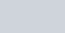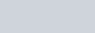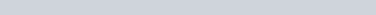# Lecture in Area Problem and the Riemann Sum Part 3

(Last Updated On: March 21, 2020)I will discuss here an important topic which I pulled out from the Area Problem and the Riemann Sum series Part I. As I said, I don’t want that post to be very long. In case you skip that part, follow the link above to read and understand the first part. When you pass the subtopic about “Options in choosing the point xi or the height of the Rectangle” click the link to bring you back here.
In Calculus, It is very important to study and understand clearly the Area Problem because it will give us one of the interpretations of a definite integral that will lead us to the definition of the definite integral. So I decided to make a separate post to elaborate the techniques of estimating the area under a curve.

### Area of the Region Between the Function and the x-axis

Again, we are going to assume that we’ve got a function f(x) that is positive on some interval [a,b]. What we want to do is determine the area of the region between the function and the x-axis.
For example, determine the area between f(x) = x2 + 1 on [0,2]. We are going to determine the area of the shaded region below.Seems a hard task to do but from what we learned on the first part of the series if we are going to enclose the shaded region with a rectangle we can estimate the area by dividing up the interval into n subintervals each of width,Now, in each interval we have the width and we have the height which is is given by the function value at a specific point in the interval. We can then find the area of each of these rectangles, add them up and this will be an estimate of the area. For better understanding keep on reading.

### Using the value at the Right Endpoint

This is the first technique or option that we are going to use for estimating the area. If we divide up the interval into 4 subintervals and use the function value at the right endpoint of each interval to define the height of the rectangle. This gives,By simply looking at the figure, we can conclude that by choosing the height as we did each of the rectangles will over estimate the area since each rectangle takes in more area than the graph each time. Let’s continue to estimate the area. The width of each of the rectangles is ½ [(b-a)/n = (2-0)/4]. The height of each rectangle is determined by the function value at the right endpoint and so the height of each rectangle is nothing more that the function value at the right endpoint. Here is the estimated area.### Using the value at the Left Endpoint

This is our second option. We could have taken the rectangle heights to be the function value at the left endpoint. Using the left endpoints as the heights of the rectangles will give the following graph and estimated area.In this case we can clearly see that our estimation will be an underestimation since each rectangle misses some of the area each time.

### Using the value at Midpoint

The last option is the common point for getting the heights of the rectangles that is often more accurate. Instead of using the right or left endpoints of each sub interval we could take the midpoint of each subinterval as the height of each rectangle. Here is the graph for this case.So, it looks like each rectangle will over and under estimate the area. This means that the approximation this time should be much better than the previous two choices of points. Here is the estimation for this case.We’ve now got three estimates. For comparison’s sake the exact area isSo, both the right and left endpoint estimation did not do all that great of a job at the estimation. The midpoint estimation however did quite well.

### Careful! Do not Draw any Conclusion yet

Be careful to not draw any conclusion about how choosing each of the points will affect our estimation.
• In this case, because we are working with an increasing function choosing the right endpoints will overestimate and choosing left endpoint will underestimate.
• If we were to work with a decreasing function we would get the opposite results. For decreasing functions the right endpoints will underestimate and the left endpoints will overestimate.
• Also, if we had a function that both increased and decreased in the interval we would, in all likelihood, not even be able to determine if we would get an overestimation or underestimation.

### Easiest Way to Get Better Approximation

The three methods is quite satisfying because none of the estimations above really did a great of a job at estimating the area. So we want a better approximation. To this, we could try to find a different point to use for the height of each rectangle but that would be useless and there wouldn’t be any guarantee that the estimation would be in fact be better. So what we are looking is the best way for getting better approximations that would work for any function we would chose to work with whether increasing function or decreasing function.
Let’s continue to work, suppose we double the number of rectangles that we used and see what happens. Below are the graphs showing the eight rectangles and the estimations for each of the three choices for rectangle heights that we used above.Here are the area estimations for each of these cases.Now, after learning all these methods of approximating the area of a curve what can you conclude? I know you already get it. The more rectangles we used the much better approximation we get.
Accordingly, the best way to get better approximation is to take more rectangles or to increase n subintervals.

### Back to Part I of the series

At this point, You are ready to continue learning the concept and the relation of Riemann Sum to Definite Integral. Kindly proceed to toward the end of part I series.

credit: Paul Dawkins, Lamar University©2013 www.PinoyBIX.com